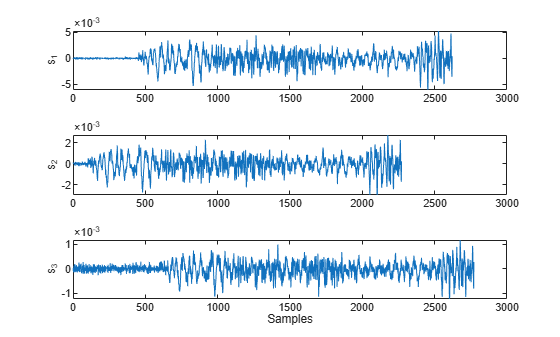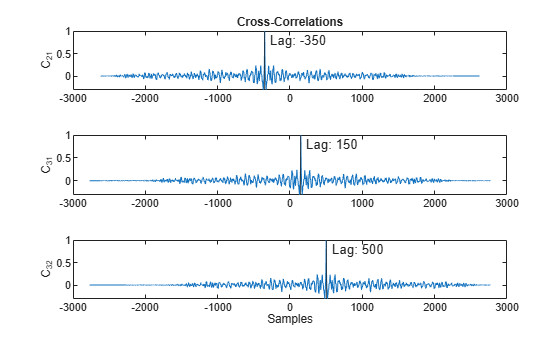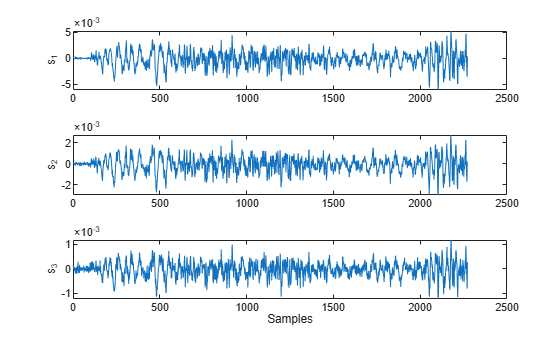# Align Signals Using Cross-Correlation

Many measurements involve data collected asynchronously by multiple sensors. If you want to integrate the signals and study them in tandem, you have to synchronize them. Use `xcorr` for that purpose.

For example, consider a car crossing a bridge. The vibrations it produces are measured by three identical sensors located at different spots. The signals have different arrival times.

Load the signals into the MATLAB® workspace and plot them.

```load relatedsig ax(1) = subplot(3,1,1); plot(s1) ylabel('s_1') axis tight ax(2) = subplot(3,1,2); plot(s2) ylabel('s_2') axis tight ax(3) = subplot(3,1,3); plot(s3) ylabel('s_3') axis tight xlabel('Samples') linkaxes(ax,'x')```Compute the cross-correlations between the three pairs of signals. Normalize them so their maximum value is 1.

```[C21,lag21] = xcorr(s2,s1); C21 = C21/max(C21); [C31,lag31] = xcorr(s3,s1); C31 = C31/max(C31); [C32,lag32] = xcorr(s3,s2); C32 = C32/max(C32);```

The locations of the maximum values of the cross-correlations indicate time leads or lags.

```[M21,I21] = max(C21); t21 = lag21(I21); [M31,I31] = max(C31); t31 = lag31(I31); [M32,I32] = max(C32); t32 = lag31(I32);```

Plot the cross-correlations. In each plot display the location of the maximum.

```subplot(3,1,1) plot(lag21,C21,[t21 t21],[-0.5 1],'r:') text(t21+100,0.5,['Lag: ' int2str(t21)]) ylabel('C_{21}') axis tight title('Cross-Correlations') subplot(3,1,2) plot(lag31,C31,[t31 t31],[-0.5 1],'r:') text(t31+100,0.5,['Lag: ' int2str(t31)]) ylabel('C_{31}') axis tight subplot(3,1,3) plot(lag32,C32,[t32 t32],[-0.5 1],'r:') text(t32+100,0.5,['Lag: ' int2str(t32)]) ylabel('C_{32}') axis tight xlabel('Samples')````s2` leads `s1` by 350 samples; `s3` lags `s1` by 150 samples. Thus `s2` leads `s3` by 500 samples. Line up the signals by clipping the vectors with longer delays.

```s1 = s1(-t21:end); s3 = s3(t32:end); ax(1) = subplot(3,1,1); plot(s1) ylabel('s_1') axis tight ax(2) = subplot(3,1,2); plot(s2) ylabel('s_2') axis tight ax(3) = subplot(3,1,3); plot(s3) ylabel('s_3') axis tight xlabel('Samples') linkaxes(ax,'x')```The signals are now synchronized and ready for further processing.